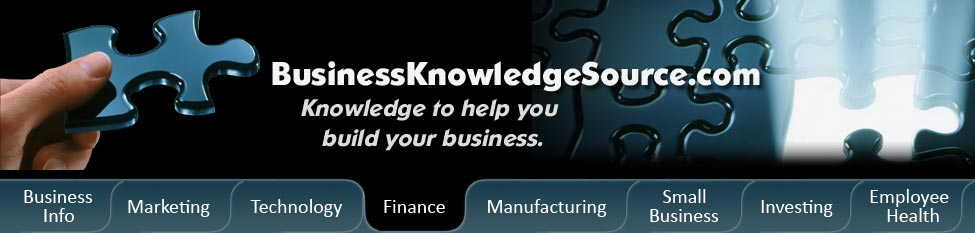# What types of assets need to be depreciatedYou've heard a lot about asset depreciation, but you don't know which of your many different types of assets need to be depreciation.Certain assets have to be depreciated, while others can't be.

Assets that can be depreciated

Continue reading "What types of assets need to be depreciated"

# What is sum of the years digits depreciationSum of the year's digits depreciation is a method of calculating the depreciation of an asset over the years.Sum of the year's digits falls under the category of accelerated depreciation methods, as opposed to straight-line depreciation.

Accelerated depreciation methods are considered to be more conservative calculations of depreciation and also more accurate methods of depreciation calculation.Accelerated depreciation calculation methods assume and rest on the idea that an asset will lose value more quickly at the beginning of its useful life as opposed to losing value at a steady rate throughout its depreciable life.Another method of calculating depreciation that falls under accelerated depreciation is double-declining balance depreciation, or just plain declining balance depreciation.

Continue reading "What is sum of the years digits depreciation"

# What are the methods of calculating depreciationThere are several different methods of calculating depreciation; these methods fall into two general categories, known as straight-line depreciation and accelerated depreciation.

Depreciation is an idea used in finance, economics, and accounting.Depreciation stems from the fact that all assets that have a measurable life-something like a car, machinery, and so on (as opposed to land)-will continue to lose value as time goes on.

Continue reading "What are the methods of calculating depreciation"

# How to make up for depreciation

Introduction

Depreciation is the reduction in value of any of your company's fixed assets.For example, the vehicles that you own or even the buildings may depreciate from year to year.In business, there is really no such thing as making up for depreciation.You can't make up for the fact that your work truck gets older every year.What you can do to try to make up for that loss is to use the loss as a tax write off.

Instructions

Difficulty:medium easy

Steps:

Continue reading "How to make up for depreciation"

# How to account for depreciation on the books

Introduction

Depreciation is the natural reduction in value of any of your company's fixed assets.For example, your vehicles and your computers are worth less every year, or they depreciate every year.If you are in charge of bookkeeping, you will need to account for that depreciation.The reason you need to account for it is that if you enter in \$20,000 on an asset sheet for a new vehicle, the amount that the vehicle is actually worth will change every year.If you don't account for depreciation, in ten years, that vehicle will still look like \$20,000 asset on your books.If the vehicle is only worth \$2000 by that time, it is misleading and wrong to have the car listed as a \$20,000 asset.

Instructions

Difficulty:medium easy

Continue reading "How to account for depreciation on the books"

# How to make up for depreciation

Introduction

Depreciation is the reduction in value of any of your company's fixed assets.For example, the vehicles that you own or even the buildings may depreciate from year to year.In business, there is really no such thing as making up for depreciation.You can't make up for the fact that your work truck gets older every year.What you can do to try to make up for that loss is to use the loss as a tax write off.

Instructions

Difficulty:medium easy

Continue reading "How to make up for depreciation"

# How to account for depreciation on the books

Introduction

Depreciation is the natural reduction in value of any of your company's fixed assets.For example, your vehicles and your computers are worth less every year, or they depreciate every year.If you are in charge of bookkeeping, you will need to account for that depreciation.The reason you need to account for it is that if you enter in \$20,000 on an asset sheet for a new vehicle, the amount that the vehicle is actually worth will change every year.If you don't account for depreciation, in ten years, that vehicle will still look like \$20,000 asset on your books.If the vehicle is only worth \$2000 by that time, it is misleading and wrong to have the car listed as a \$20,000 asset.

Continue reading "How to account for depreciation on the books"Subscribe to our free newsletter and get our "How To Get More Leads" course free via email. Just enter your first name and email address below to subscribe.
First Name *
Email *

Marketing and Sales
Technology
Finance
Manufacturing